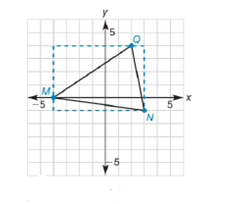Chapter 10.1, Problem 33EElementary Geometry For College St...

7th Edition
Alexander + 2 others
ISBN: 9781337614085

Solutions

Chapter
SectionElementary Geometry For College St...

7th Edition
Alexander + 2 others
ISBN: 9781337614085
Textbook Problem

The triangle that has vertices at M - 4 ,   0 ,   N 3 ,   - 1 ,     a n d   Q ( 2 ,   4 ) has been boxed in, as shown. Find the area of ∆ M N Q .Exercises 33, 34.

To determine

To find:

The area of MNQ and the vertices are M-4, 0, N3, -1, and Q(2, 4).

Explanation

Given vertices are,

M-4, 0, N3, -1, and Q(2, 4)

Given figure is,

Sketch the points and draw the triangle in the graph as shown in figure,

Base of the triangle can be determined by subtracting x-coordinates of MN

Base MN=3--4=7

Height of the triangle can be determined by subtracting

Still sussing out bartleby?

Check out a sample textbook solution.

See a sample solution

The Solution to Your Study Problems

Bartleby provides explanations to thousands of textbook problems written by our experts, many with advanced degrees!

Get Started

Solve the equations in Exercises 112 for x (mentally, if possible). x1=cx+d(c1)

Finite Mathematics and Applied Calculus (MindTap Course List)

Fill in each blank: 54in.=ft

Elementary Technical Mathematics

1. Convert the constraint inequalities to equations containing slack variables.

Mathematical Applications for the Management, Life, and Social Sciences

In Exercises 1-4, simplify the expression by factoring. x5+9x4x2

Calculus: An Applied Approach (MindTap Course List)

What is the future value of an annuity? Give an example.

Finite Mathematics for the Managerial, Life, and Social Sciences